# Four long parallel wires each carry a 2.0 A current in the same direction. The wires...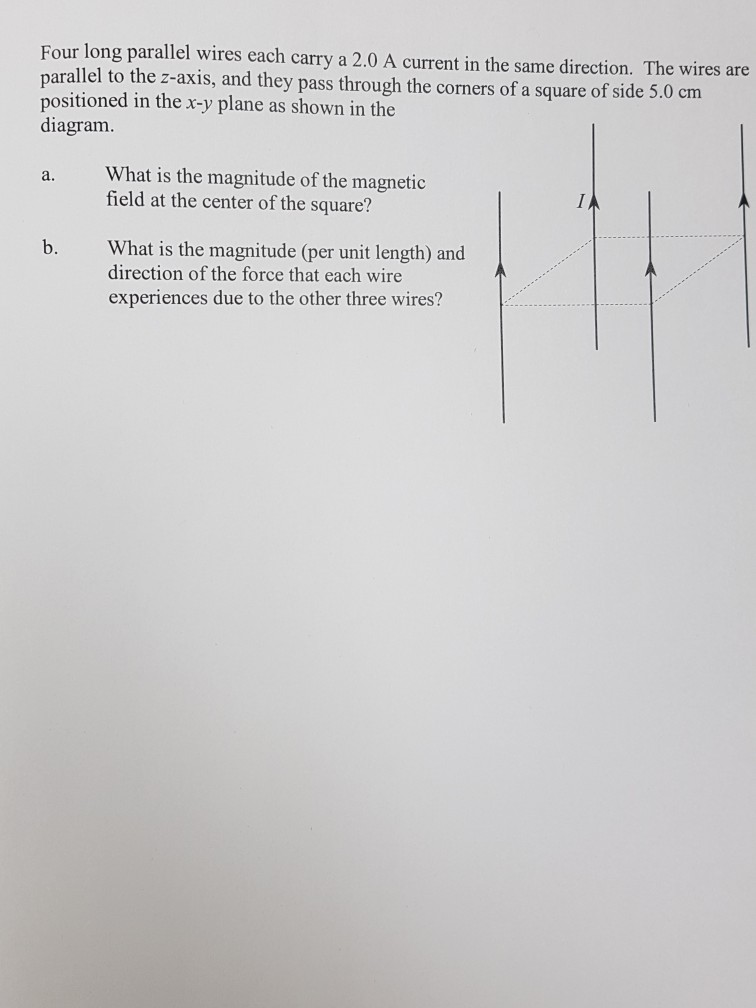Four long parallel wires each carry a 2.0 A current in the same direction. The wires are parallel to the z-axis, and they pass through the corners of a square of side 5.0 cm in the x-y plane as shown in the diagram. What is the magnitude of the magnetic field at the center of the square? a. b. What is the magnitude (per unit length) and direction of the force that each wire experiences due to the other three wires?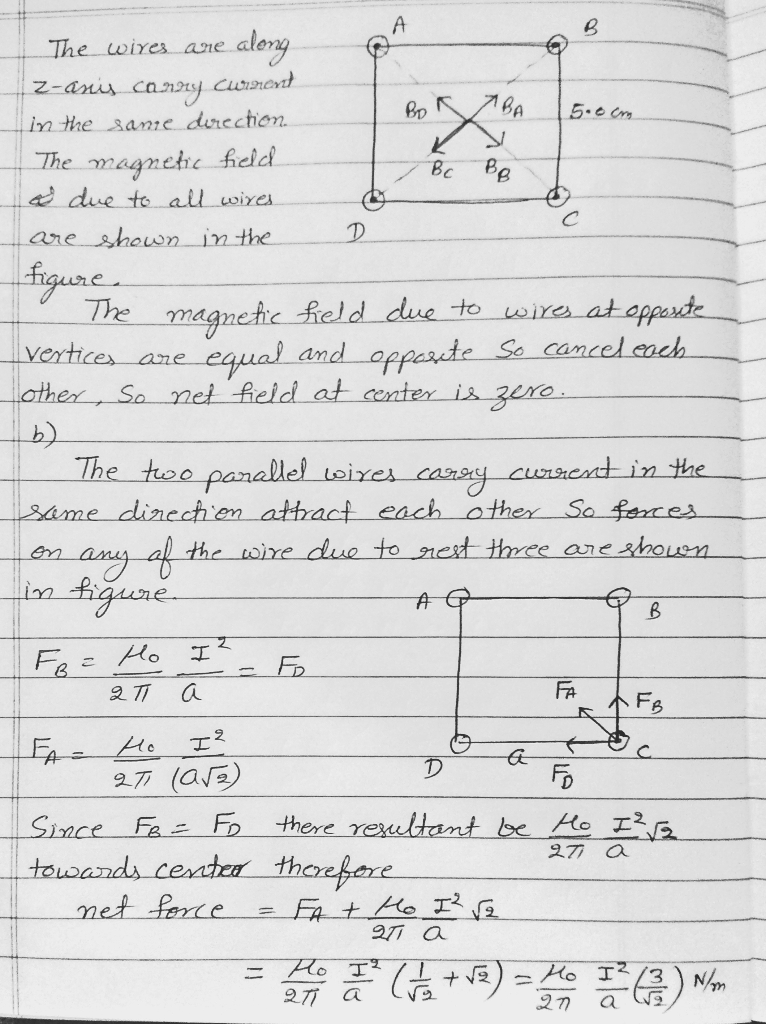because of symmetry each of the wire experience same force directed towards center.

In case of any doubt please comment

#### Earn Coin

Coins can be redeemed for fabulous gifts.

Similar Homework Help Questions
• ### Four long parallel wires each carry 2.0 A in the same direction. They are parallel to...

Four long parallel wires each carry 2.0 A in the same direction. They are parallel to the z-axis, and they pass through the corners of a square of side 4.0 cm positioned in the x-y plane. What net magnetic field does one of the wires experience due to the other wires?

• ### Four long parallel wires each carry a current of 1.0 A. The current direction is out...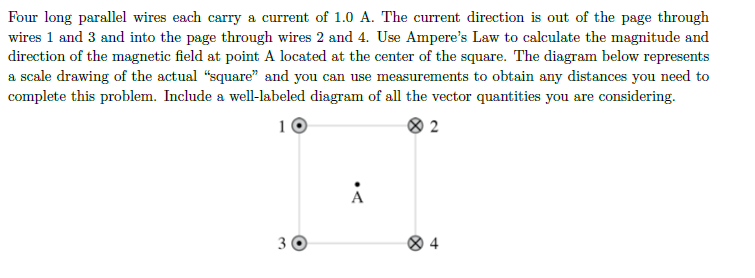Four long parallel wires each carry a current of 1.0 A. The current direction is out of the page through wires 1 and 3 and into the page through wires 2 and 4. Use Ampere's Law to calculate the magnitude and direction of the magnetic field at point A located at the center of the square. The diagram below represents a scale drawing of the actual "square" and you can use measurements to obtain any distances you need to complete...

• ### Two parallel wires carry electric current in the same direction as shown in the figure to...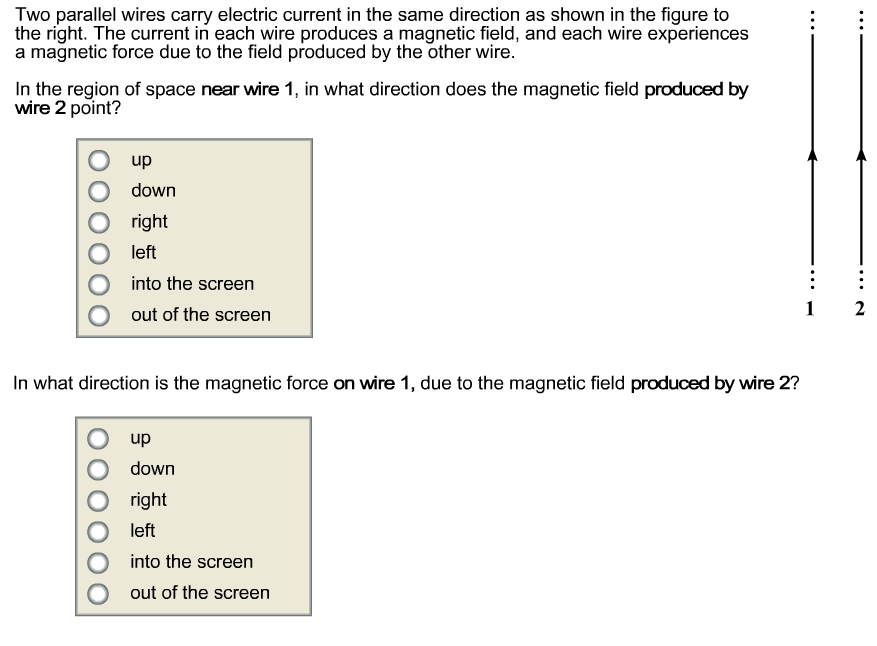Two parallel wires carry electric current in the same direction as shown in the figure to the right. The current in each wire produces a magnetic field, and each wire experiences a magnetic force due to the field produced by the other wire In the region of space near wire 1, in what direction does the magnetic field produced by wire 2 point? O up O down O right O left into the screen O out of the screen In...

• ### ch.28 12

Four long parallel wires each carry 2.0 A in the same direction. They are parallel to the z-axis, and they pass through the corners of a square of side 4.0 cmpositioned in the x-y plane. What magnetic field does one of the wires experience due to the other wires?

• ### Four long parallel wires pass through the corners of a square with side 0.62 m. All...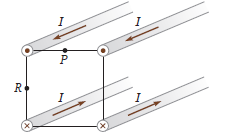Four long parallel wires pass through the corners of a square with side 0.62 m. All four wires carry the same magnitude of current I = 24.1 A in the directions indicated. Find the magnetic field at point P, the midpoint of the top side of the square. Four long parallel wires pass through the corners of a square with side 0.62 m. All four wires carry the same magnitude of current I = 24.1 A in the directions indicated....

• ### 9. Four wires are running parallel at the corners of a square. The wires run parallel to the x ax...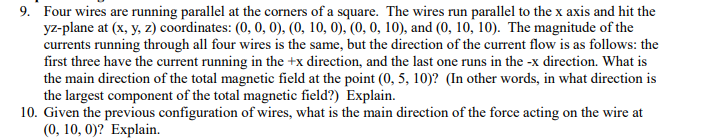9. Four wires are running parallel at the corners of a square. The wires run parallel to the x axis and hit the yz-plane at (x, y, z) coordinates: (0, 0, 0), (0, 10, 0), (0, 0, 10), and (0, 10, 10). The magnitude of the currents running through all four wires is the same, but the direction of the current flow is as follows: the first three have the current running in the +x direction, and the last one...

• ### 3) Three long parallel conductors carry equal currents of I= 5 Amperes. The wires are separated...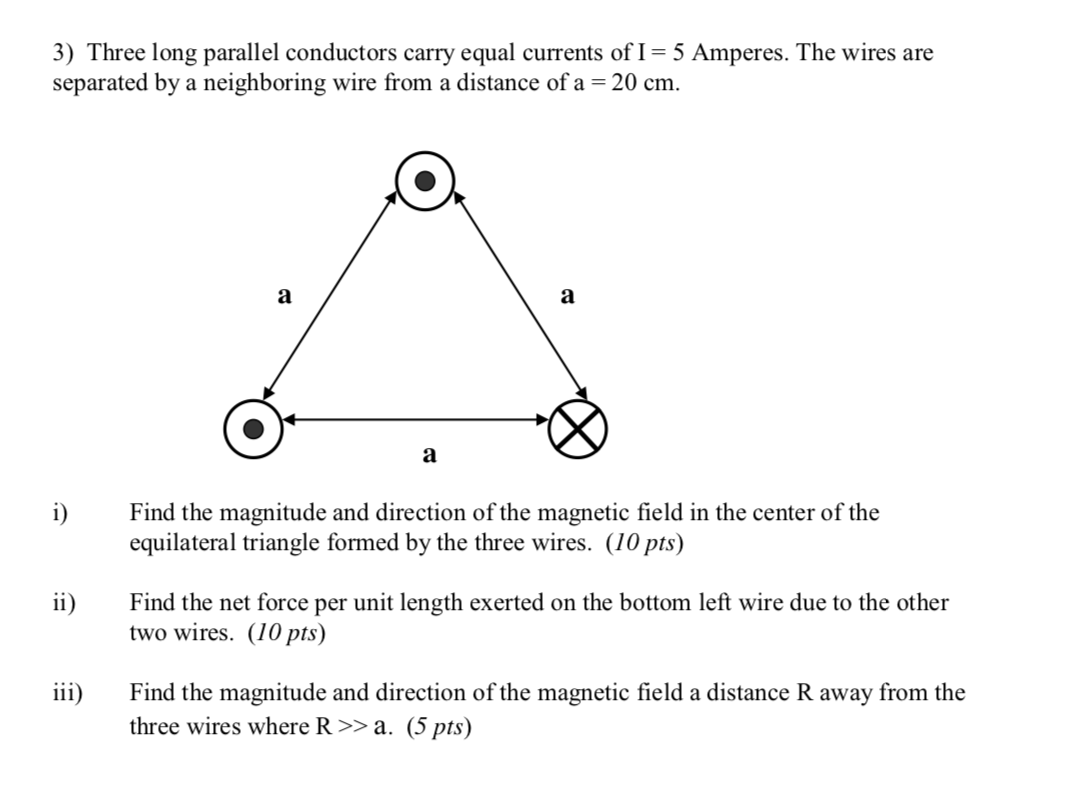3) Three long parallel conductors carry equal currents of I= 5 Amperes. The wires are separated by a neighboring wire from a distance of a = 20 cm. a a а i) Find the magnitude and direction of the magnetic field in the center of the equilateral triangle formed by the three wires. (10 pts) ii) Find the net force per unit length exerted on the bottom left wire due to the other two wires. (10 pts) iii) Find the...

• ### 2 long straight wires run parallel to each other, and carry currentS flowing in the same...

2 long straight wires run parallel to each other, and carry currentS flowing in the same direction. Wire 1 carries a current of 1.2A,and wire 2 carries a current of 1.6A. The wires are 15 cm apart.a) What is the magnetic field from wire 1 at the location of wire 2?b)What is the magnetic force per unit length felt by wire 2? C)What is the magnetic force per unit length felt by wire 1?

• ### 2 long straight wires run parallel to each other, and carry currentS flowing in the same...

2 long straight wires run parallel to each other, and carry currentS flowing in the same direction. Wire 1 carries a current of 1.2A,and wire 2 carries a current of 1.6A. The wires are 15 cm apart.a) What is the magnetic field from wire 1 at the location of wire 2?b)What is the magnetic force per unit length felt by wire 2? C)What is the magnetic force per unit length felt by wire 1?

• ### Two long straight wires run parallel to each other, and carry currents flowing in the same...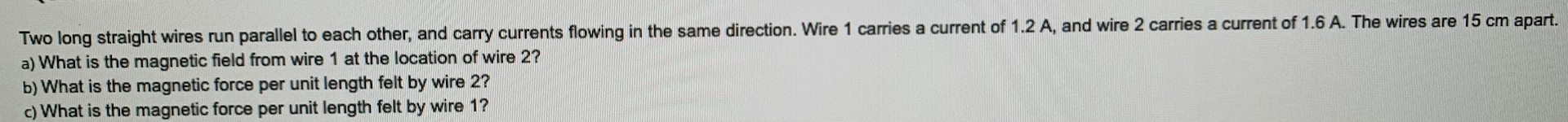Two long straight wires run parallel to each other, and carry currents flowing in the same direction. Wire 1 carries a current of 1.2 A, and wire 2 carries a current of 1.6 A. The wires are 15 cm apart. a) What is the magnetic field from wire 1 at the location of wire 2? b) What is the magnetic force per unit length felt by wire 2? c) What is the magnetic force per unit length felt by wire...